## Example Questions

2 Next →

### Example Question #11 : How To Find The Nth Term Of An Arithmetic Sequence

An arithmetic sequence begins as follows: 14, 27, 40...

What is the first four-digit integer in the sequence?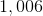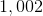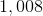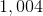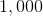Explanation:

Given the first two terms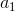and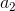, the common differenceis equal to the difference: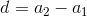Setting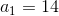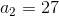: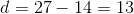Theth term of an arithmetic sequence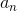can be found by way of the formula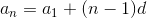Since we are looking for the first four-digit whole number - equivalently, the first number greater than or equal to 1,000: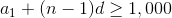Settingand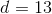and solving for: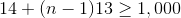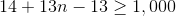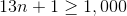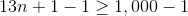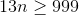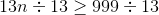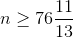Therefore, the 77th term, or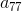, is the first element in the sequence greater than 1,000. Substituting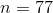, andin the rule and evaluating: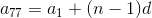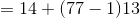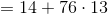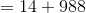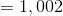,

the correct choice.

2 Next →